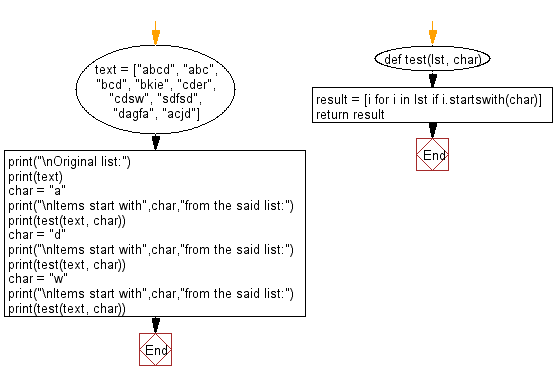﻿ Python: Find the items start with specific character from a given list - w3resource# Python: Find the items start with specific character from a given list

## Python List: Exercise - 70 with Solution

Write a Python program to find the items starts with specific character from a given list.

Sample Solution:-

Python Code:

``````def test(lst, char):
result = [i for i in lst if i.startswith(char)]
return result
text = ["abcd", "abc", "bcd", "bkie", "cder", "cdsw", "sdfsd", "dagfa", "acjd"]
print("\nOriginal list:")
print(text)
char = "a"
print(test(text, char))
char = "d"
print(test(text, char))
char = "w"
print(test(text, char))
```
```

Sample Output:

```Original list:
['abcd', 'abc', 'bcd', 'bkie', 'cder', 'cdsw', 'sdfsd', 'dagfa', 'acjd']

['abcd', 'abc', 'acjd']

['dagfa']

[]
```

Flowchart:## Visualize Python code execution:

The following tool visualize what the computer is doing step-by-step as it executes the said program:

Python Code Editor:

Have another way to solve this solution? Contribute your code (and comments) through Disqus.

What is the difficulty level of this exercise?

Test your Python skills with w3resource's quiz

﻿

## Python: Tips of the Day

```print(2_000_000)
```2000000